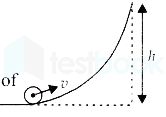Question

# A small object of uniform density rolls up a curved surface with an initial velocity $v$. It reaches up to a maximum height of $\frac{3{v}^{2}}{4g}$ with respect to the initial position. The object is a

Open in App
Solution

## Step1: Given dataThe initial velocity of the body is $u=v$.The maximum height of the body on the curved surface is $H=\frac{3{v}^{2}}{4g}$.DiagramStep2: Conservation of energyFrom the concept of conservation of energy, the sum of kinetic and potential energy is conserved in every point of motion. i.e., $\frac{1}{2}m{v}^{2}+\frac{1}{2}I{\omega }^{2}=mgh$The kinetic energy of a body moving with a velocity is ${K}_{t}=\frac{1}{2}m{v}^{2}$ and the kinetic energy due to rotation is $\frac{1}{2}I{\omega }^{2}$, where, m is the mass of the body, I is the moment of inertia, and $\omega$ is the angular velocity of the body.The potential energy of a body at height h is $mgh$, and g is the acceleration due to gravity.Step4: Finding the moment of inertia We know from the conservation of kinetic energy, that the sum of kinetic and potential energy is conserved at $h=0$ and $h=h$.So, $\frac{1}{2}m{v}^{2}+\frac{1}{2}I{\omega }^{2}=mgh$.$or\frac{1}{2}m{v}^{2}+\frac{1}{2}I{\omega }^{2}=mgh.\phantom{\rule{0ex}{0ex}}or\frac{1}{2}m{v}^{2}+\frac{1}{2}I{\left(\frac{v}{R}\right)}^{2}=mg\left(\frac{3{v}^{2}}{4g}\right)\left(\mathrm{sin}ce,\omega =\frac{v}{R}andh=\frac{3{v}^{2}}{4g}\right)\phantom{\rule{0ex}{0ex}}or\frac{1}{2}I{\left(\frac{v}{R}\right)}^{2}=mg\left(\frac{3{v}^{2}}{4g}\right)-\frac{1}{2}m{v}^{2}\phantom{\rule{0ex}{0ex}}or\frac{1}{2}I{\left(\frac{v}{R}\right)}^{2}=\frac{3m{v}^{2}}{4}-\frac{1}{2}m{v}^{2}\phantom{\rule{0ex}{0ex}}or\frac{1}{2}I{\left(\frac{v}{R}\right)}^{2}=\frac{1}{4}m{v}^{2}\phantom{\rule{0ex}{0ex}}orI=\frac{\frac{1}{4}m{v}^{2}}{\frac{1}{2}{\left(\frac{v}{R}\right)}^{2}}=\frac{1}{2}\frac{m{v}^{2}.{R}^{2}}{{v}^{2}}=\frac{1}{2}m{R}^{2}\phantom{\rule{0ex}{0ex}}orI=\frac{1}{2}m{R}^{2}.$Therefore, the moment of inertia of the body is $I=\frac{1}{2}m{R}^{2}$, this is the moment of inertia of a disc about an axis passing through the center perpendicular to the plane. So, the object is disc.Suggest Corrections0Similar questionsExplore more